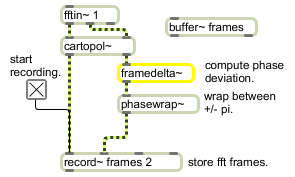# framedelta~

Compute phase deviation between successive FFT frames

## Description

framedelta~ computes a running phase deviation by subtracting values in each position of its previously received signal vector from the current signal vector. When used inside a pfft~ object, it keeps a running phase deviation of the FFT because the FFT size is equal to the signal vector size.

None.

## Messages

 signal The input on which the deviation will be computed.

## Output

signal: For each signal vector, the first sample of its output will be the first sample in the current signal vector minus the first sample in the previous signal vector, the second sample of its output will be the second sample in the current signal vector minus the second sample in the previous signal vector, and so on.

## Examplesframedelta~ computes the difference between successive frames of FFT data